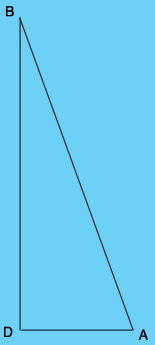SEARCH HOMEMath Central Quandaries & QueriesQuestion from mohammad, a student: Two boats leave the same dock at the same time,one traveling due east at 10 mph and the other due north at 24mph,how many miles apart are the boats after 3hours ?Hi,

I drew a diagram of the positions of the boats after 3 hours (not to scale). D is the dock, A is the position of the slower boat and B is the position of the faster boat.The slower boat travels at 10 mph so what is the distance from D to A? What is the distance from D to B? You want the distance from A to B. Since angle BDA is a right angle Pythagoras can help.

PennyMath Central is supported by the University of Regina and the Imperial Oil Foundation.